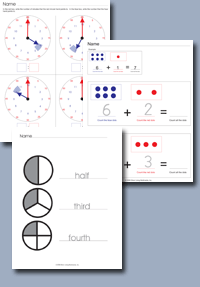﻿ Common Core Standards - MathAdaptive Worksheets

Helping Every Child to Succeed

toll-free (888) 777-0876
fax (888) 777-0875

## Draw and identify lines and angles, and classify shapes by properties of their lines and angles.

• CCSS.Math.Content.4.G.A.1 Draw points, lines, line segments, rays, angles (right, acute, obtuse), and perpendicular and parallel lines. Identify these in two-dimensional figures.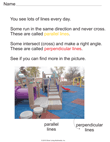Parallel and Perpendicular Lines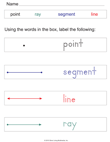Label Lines, Segments and Rays
• CCSS.Math.Content.4.G.A.2 Classify two-dimensional figures based on the presence or absence of parallel or perpendicular lines, or the presence or absence of angles of a specified size. Recognize right triangles as a category, and identify right triangles.Identify Right Triangles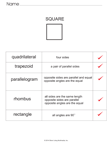Quadrilateral Comparison Chart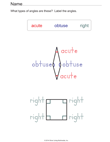Identify Types of Angles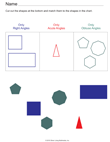Sort Shapes By Angle Type
• CCSS.Math.Content.4.G.A.3 Recognize a line of symmetry for a two-dimensional figure as a line across the figure such that the figure can be folded along the line into matching parts. Identify line-symmetric figures and draw lines of symmetry.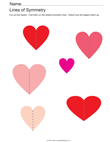Symmetric HeartsSymmetric Arrows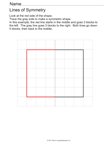Lines of Symmetry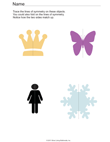Symmetric FiguresDraw The Other Half